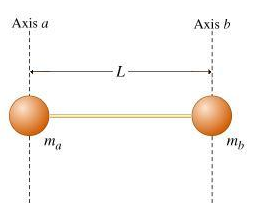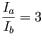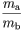# Problem: Ball a, of mass ma, is connected to ball b, of mass mb, by a massless rod of length L. The two vertical dashed lines in the figure, one through each ball, represent two different axes of rotation, axes a and b. These axes are parallel to each other and perpendicular to the rod. The moment of inertia of the two-mass system about axis a Ia, and the moment of inertia of the system about axis b is Ib It is observed that the ratio of  Ia to Ib is equal to 3:(a) Assume that both balls are point-like; that is, neither has any moment of inertia about its own center of mass. Q. Find the ratio of the masses of the two balls. = ? (b) Find da, the distance from ball A to the system's center of mass.(Express your answer in terms of L ,the length of the rod.)

###### FREE Expert Solution
86% (6 ratings)View Complete Written Solution
###### Problem DetailsBall a, of mass ma, is connected to ball b, of mass mb, by a massless rod of length L. The two vertical dashed lines in the figure, one through each ball, represent two different axes of rotation, axes a and b. These axes are parallel to each other and perpendicular to the rod. The moment of inertia of the two-mass system about axis a Ia, and the moment of inertia of the system about axis b is Ib It is observed that the ratio of  Ia to Ib is equal to 3:(a) Assume that both balls are point-like; that is, neither has any moment of inertia about its own center of mass. Q. Find the ratio of the masses of the two balls.= ?

(b) Find da, the distance from ball A to the system's center of mass.

(Express your answer in terms of L ,the length of the rod.)